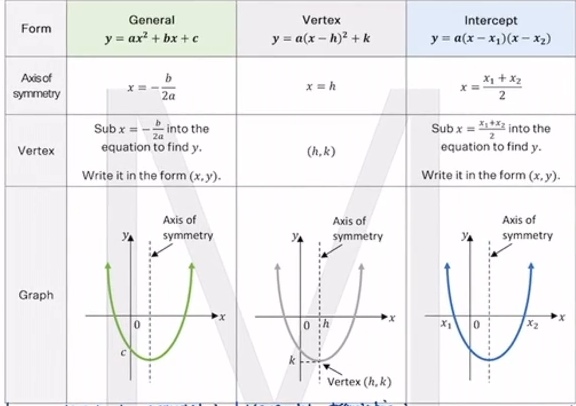# In a Nutshell: Parabola Forms

## part of the In a Nutshell series on Adrian’s Study Club

### Factored form `y=a(x-x_1)(x-x_2)`

Given:

1. 2 x-ints
2. One other point `P(x,y)

### Vertex Form `y=a(x-h)^2 + k`

Given:

1. Vertex `V(h,k)`
2. `P(x, y)`

### General Form `y=ax^2 + bx + c`

Given:

1. 3 points

OR

1. 2 points
2. y-int where `y-int = c`

OR

1. Axis of symmetry `x=-(b/2a)
2. 2 other points

Form General `y = ax^-2 + bx + c` Vertex `y =a (x-h)^2 + k` Intercept `y=(x-x_1)(x-x_2)`
Axis of symmetry `x = -(b/2a)` `x = h` `x=((x_1 + x_2)/2)`
Vertex Sub `x = -(b/2a)` into the equation to find `y`. `(h, k)` Sub `x=((x_1 + x_2)/2)` into the equation to find y.
Form Vertical Translation `y = ax^2 + c` Horizontal translation `y=a(x-h)^2`
Axis of symmetry `x=0` (y-axis) `x=h`
Vertex `V(O,c)` `V(h,0)`